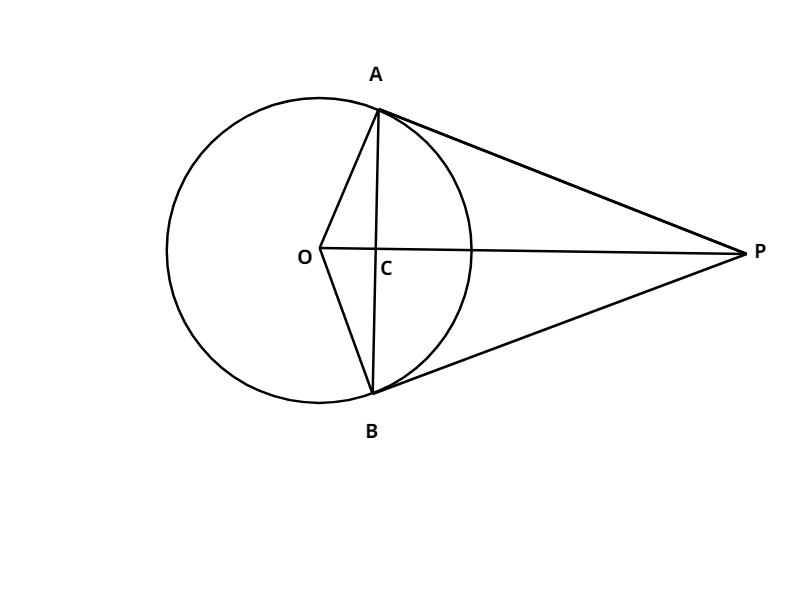QUESTION

# From an external point P two tangents PA and PB are drawn to the circle with center O. Prove that OP is a perpendicular bisector of AB.

Hint- In this particular type of question we need to draw a diagram to develop a clear picture of what we have to do to prove OP as the perpendicular bisector of AB. First we have to prove triangle PAC and triangle PBC congruent and then using CPCT (Corresponding Parts of Congruent Triangles) to prove OP as the bisector of AB. Further we need to prove angle ACB equal to angle PCB equal to $90^\circ$ to get the desired result.Let OP intersect AB at C,
In $\vartriangle PAC{\text{ and }}\vartriangle {\text{PBC}}$, we have
PA = PB (Tangents on a circle from an external point are equal in length)
$\angle APC = \angle BPC$ (PA and PB are equally inclined to OP)
and PC = PC ( Common)
$\therefore {\text{ }}\vartriangle PAC \cong \vartriangle PBC$ (by SAS congruence criteria)
$\Rightarrow$AC = BC
And $\angle ACP = \angle BCP$
(by using CPCT)
$\begin{gathered} \angle ACP + \angle BCP = 180^\circ \\ \Rightarrow 2\angle ACP = 180^\circ \\ \Rightarrow \angle ACP = \angle BCP = \frac{{180^\circ }}{2} = 90^\circ \\ \end{gathered}$( sum of angles on a line is 180$^\circ$)
Hence, we can conclude that OP is the perpendicular bisector of AB as OP divides AB into two equal parts and is perpendicular to it.

Note- It is important to recall the triangle congruence rules while solving this question. Note that this question could also be done by first proving triangle PBO congruent to triangle APO proving angles APO and BPO equal and then further proving congruence of triangles ACP and PBC.